# Python 机器学习实战：根据成绩预测大学生能否被高校录取

42 篇文章 9 订阅

逻辑回归算法是用于分类的。本案例中，建立一个逻辑回归模型来预测一个学生是否被大学录取。假设你是一个大学系的管理员，你想根据两次考试的结果来决定每个申请人的录取机会。你有以前的申请人的历史数据，你可以用它作为逻辑回归的训练集。对于每一个培训例子，你有两个考试的申请人的分数和录取决定。为了做到这一点，我们将建立一个分类模型，根据考试成绩估计入学概率。

1、首先，导入库，并且读取数据集。原来数据集是 .txt 结尾的。

import numpy as np
import pandas as pd
import matplotlib.pyplot as plt

import os
path =  'LogiReg_data.txt'
pdData = pd.read_csv(path, header=None, names=['Exam 1', 'Exam 2', 'Admitted'])

pdData.head()
pdData.shape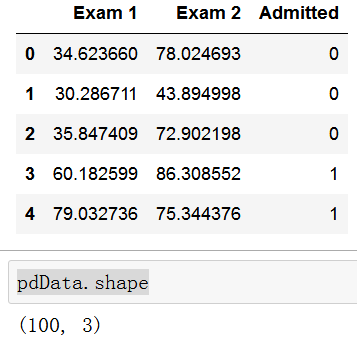2、将数据分成正负样本，利用散点图，大致看一下数据分布（不是必要步骤，而且因为数据只有两个维度，才添加了此步骤）

positive = pdData[pdData['Admitted'] == 1]

fig, ax = plt.subplots(figsize=(10,5))
ax.scatter(positive['Exam 1'], positive['Exam 2'], s=30, c='b', marker='o', label='Admitted')
ax.scatter(negative['Exam 1'], negative['Exam 2'], s=30, c='r', marker='x', label='Not Admitted')
ax.legend()
ax.set_xlabel('Exam 1 Score')
ax.set_ylabel('Exam 2 Score')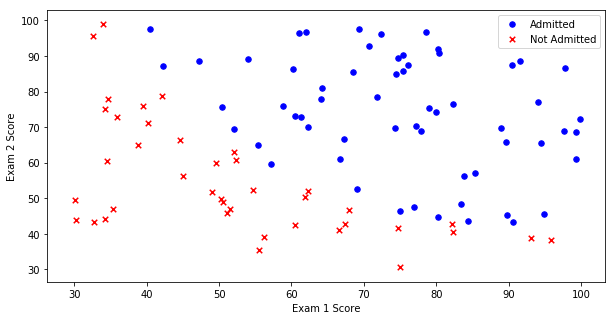3、逻辑回归

此部分，我们主要建立一个分类器：也就是求解 theta 值。然后设定阈值，根据阈值，判断是否被录取。主要步骤如下：

（1）、定义 sigmoid 函数

def sigmoid(z):
return 1 / (1 + np.exp(-z))

sigmoid 函数是将预测值（比如线性回归中的结果），映射为概率的一个函数。形式为：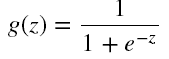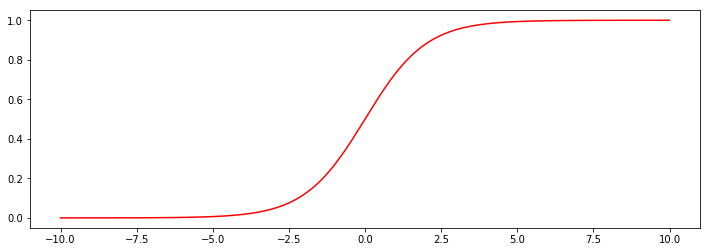（2）定义模型，也就是预测函数

def model(X, theta):
return sigmoid(np.dot(X, theta.T))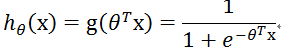将 sigmoid () 函数的自变量 z 变成上式。其中，X 是样本数据，它的每一行都是一个样本，每一列为样本的某一个特征。theta 表示参数，它是我们通过学习获得的，其中，对于每一个特征，都对应一个 theta ,即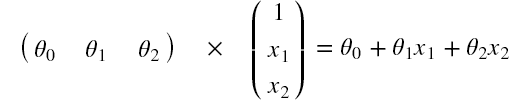pdData.insert(0, 'Ones', 1) # 在第 0 列，插入一列，名称为"Onces"，数值全为 1

# set X (training data) and y (target variable)
orig_data = pdData.as_matrix() # convert the Pandas representation of the data to an array useful for further computations
cols = orig_data.shape
X = orig_data[:,0:cols-1]
y = orig_data[:,cols-1:cols]

theta = np.zeros([1, 3])  # 初始化theta 

(3) 定义损失函数

损失函数是将对数似然函数，乘以一个负号。乘以负号是为了将求解梯度上升转换为求解梯度下降，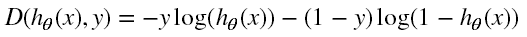这是整体的一个损失，但是，不同的样本量，总损失肯定是不同的，因此，为了确定一个统一标准，使用平均损失，即将总损失除以样本个数，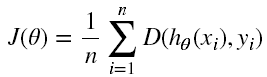def cost(X, y, theta):
left = np.multiply(-y, np.log(model(X, theta)))
right = np.multiply(1 - y, np.log(1 - model(X, theta)))
return np.sum(left - right) / (len(X))

cost(X, y, theta)

（4）梯度的计算与参数的更新

计算梯度的目的是寻找极值，确定损失函数如何进行优化，使损失函数的值越来越小。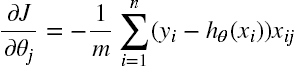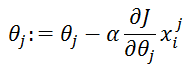我们需要通过迭代来计算梯度，然后，梯度的计算什么时候停止呢？这里有三种停止策略：

1、设置固定的迭代次数

2、设置损失函数的阈值，当达到一定阈值时，就停止迭代。

3、通过梯度的变化率来判断：设置前后两次梯度相差的阈值，如果小于该阈值，停止迭代。

STOP_ITER = 0
STOP_COST = 1

def stopCriterion(type, value, threshold):
#设定三种不同的停止策略
if type == STOP_ITER:        return value > threshold
elif type == STOP_COST:      return abs(value[-1]-value[-2]) < threshold
elif type == STOP_GRAD:      return np.linalg.norm(value) < threshold

import numpy.random
#洗牌
def shuffleData(data):
np.random.shuffle(data)
cols = data.shape
X = data[:, 0:cols-1]
y = data[:, cols-1:]
return X, y

import time

def descent(data, theta, batchSize, stopType, thresh, alpha):
#梯度下降求解

init_time = time.time()
i = 0 # 迭代次数
k = 0 # batch
X, y = shuffleData(data)
costs = [cost(X, y, theta)] # 损失值

while True:
k += batchSize  #取batch数量个数据
if k >= n:    # 这个 n 是在运行的时候指定的，为样本的个数
k = 0
X, y = shuffleData(data) #重新洗牌
theta = theta - alpha*grad # 参数更新
costs.append(cost(X, y, theta)) # 计算新的损失
i += 1

if stopType == STOP_ITER:       value = i
elif stopType == STOP_COST:     value = costs
if stopCriterion(stopType, value, thresh): break

return theta, i-1, costs, grad, time.time() - init_time

def run(data, theta, batchSize, stopType, thresh, alpha):
#import pdb; pdb.set_trace();
theta, iter, costs, grad, dur = descent(data, theta, batchSize, stopType, thresh, alpha)
fig, ax = plt.subplots(figsize=(12,4))
ax.plot(np.arange(len(costs)), costs, 'r')
ax.set_xlabel('Iterations')
ax.set_ylabel('Cost')
ax.set_title(name.upper() + ' - Error vs. Iteration')
return theta

run(orig_data, theta, n, STOP_GRAD, thresh=0.005, alpha=0.001)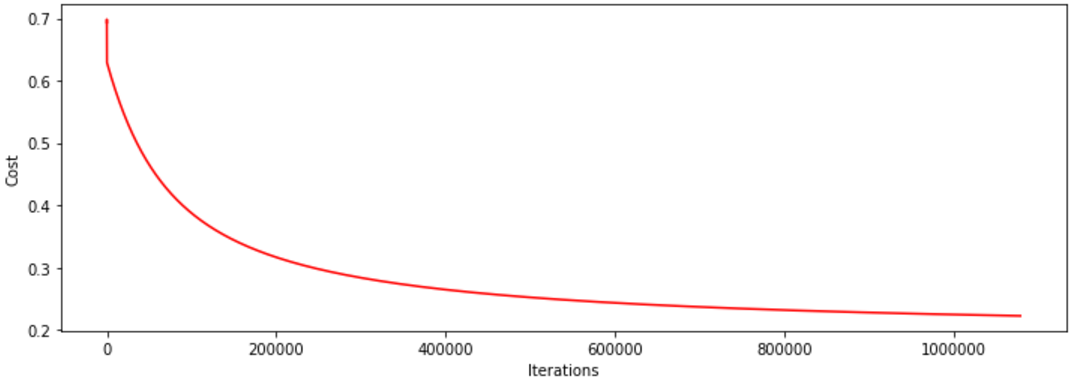run(orig_data, theta, 1, STOP_ITER, thresh=5000, alpha=0.001)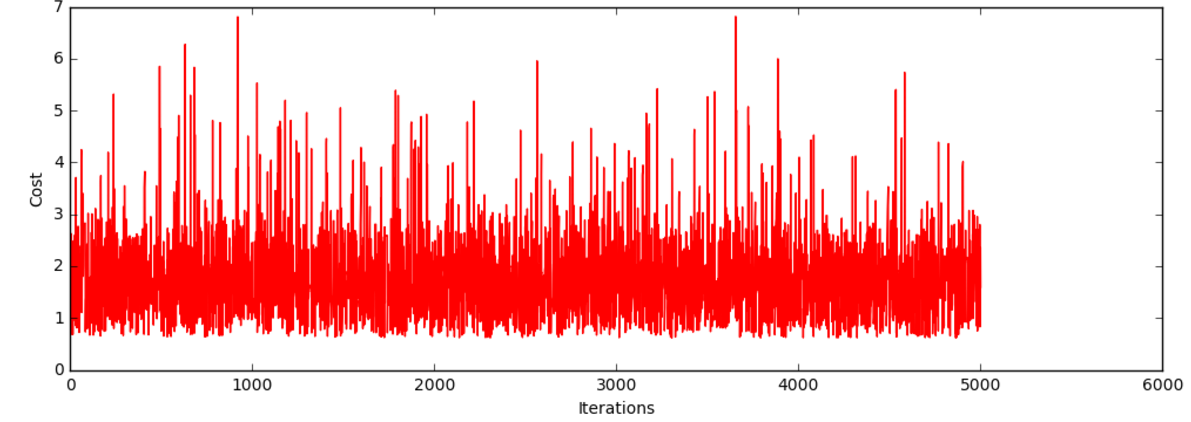（1）将学习率调小一点，情况肯定会有所改善

（2）对数据去均值化。将数据按其属性(按列进行)减去其均值，然后除以其方差。最后得到的结果是，对每个属性/每列来说所有数据都聚集在0附近，方差值为1。

from sklearn import preprocessing as pp

scaled_data = orig_data.copy()
scaled_data[:, 1:3] = pp.scale(orig_data[:, 1:3])

最终的结果是：波动不再明显，并且收敛速度加快，最终获得的损失函数的值会更小。不再一一列举实验

4、精度判断

我们训练好的模型到底好不好用，测试结果来说话：

#设定阈值
def predict(X, theta):
return [1 if x >= 0.5 else 0 for x in model(X, theta)]
scaled_X = scaled_data[:, :3]
y = scaled_data[:, 3]
predictions = predict(scaled_X, theta)
correct = [1 if ((a == 1 and b == 1) or (a == 0 and b == 0)) else 0 for (a, b) in zip(predictions, y)]
accuracy = (sum(map(int, correct)) % len(correct))
print ('accuracy = {0}%'.format(accuracy))

05-1578007-313818
11-274
08-19977
10-311763
07-10355
09-061646
12-24262
12-18373
11-20277
11-241075
06-144823
09-0241
06-25
09-02170
09-033480点击重新获取扫码支付余额充值